2018-10-17 00:18:58 qq_39736559 阅读数 311
• ###### 机器学习--线性回归数学推导视频教学

线性回归数学推导是机器学习的基石，是人工智能的算法基础， 可以被广泛的应用在在工作和学习。本节课就带领同学们从零开始一步一步的推导线性回归。课程内容包括矩阵转换、误差值分析、似然函数、小二乘、结果推导。 任务作业： 设计并实现一个三层架构的员工管理系统。要求： 1、数据库及其表自行设计，遵循数据库及表设计的一般命名规则 2、要求有管理员的登录功能及对员工信息的增删改查 3、功能的实现严格采用三层架构的设计框架，注意命名的一般规范 4、采用B/S架构，界面大方美观 5、思考下三层架构与MVC框架有什么区别 （温馨提示： 注意 作业需写在CSDN博客中，请把作业链接贴在评论区，老师会定期逐个批改~~）

3970 人正在学习 去看看 陆永剑

# KNN算法概述

KNN(k-nearest neighbor)算法属于机器学习中的有监督分类算法，主要用于分类，是最简单的机器学习算法之一顾名思义，其算法主体思想就是根据距离相近的邻居类别，来判定自己的所属类别。

# KNN算法思路

1、计算测试对象与训练集中所有对象的距离，一般采用欧式距离。
2、找出与计算对象距离最近的K个对象，作为测试对象的邻居；
3、找出这K个对象中出现频率最高的类别，该类别即为测试对象的所属类别。

# 一个简单的例子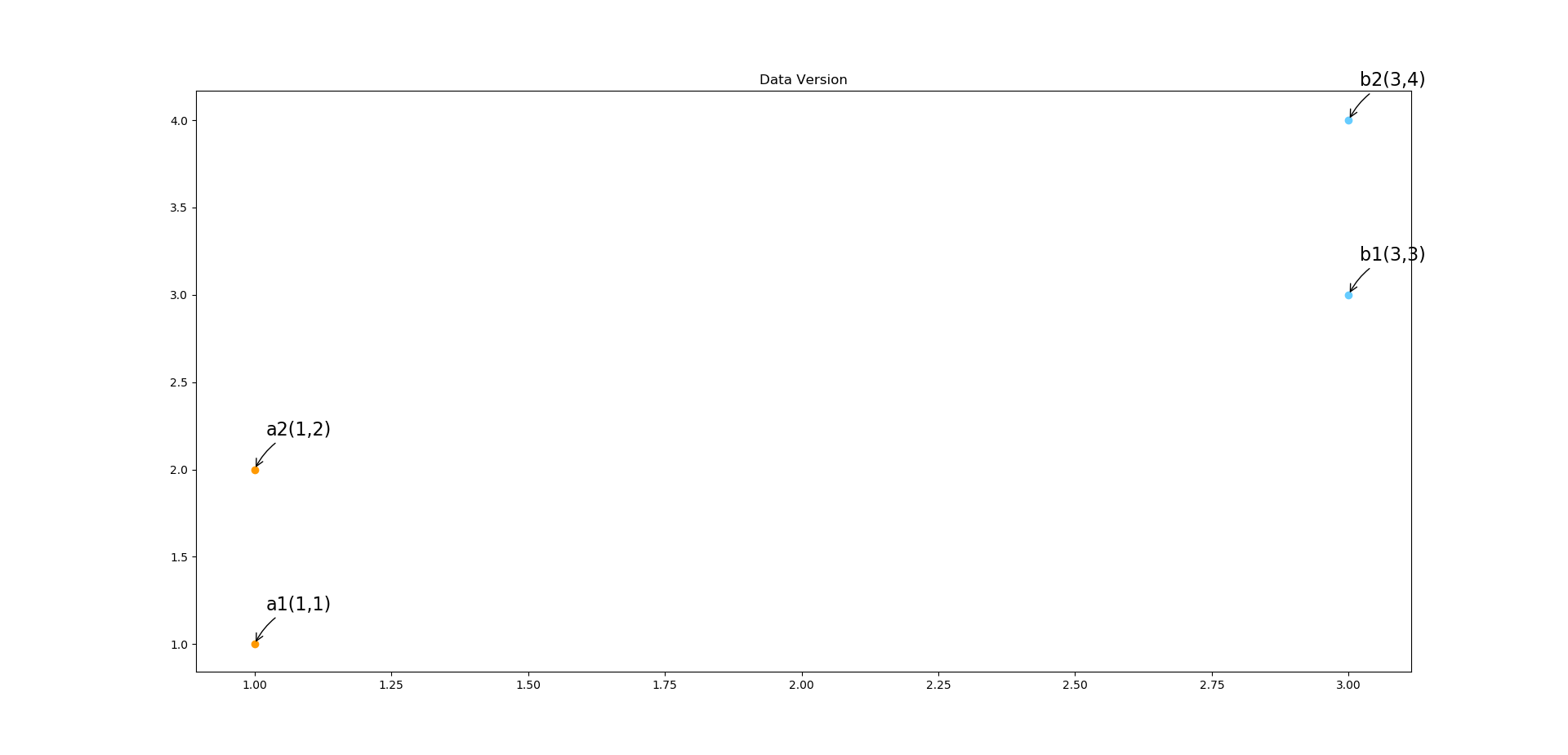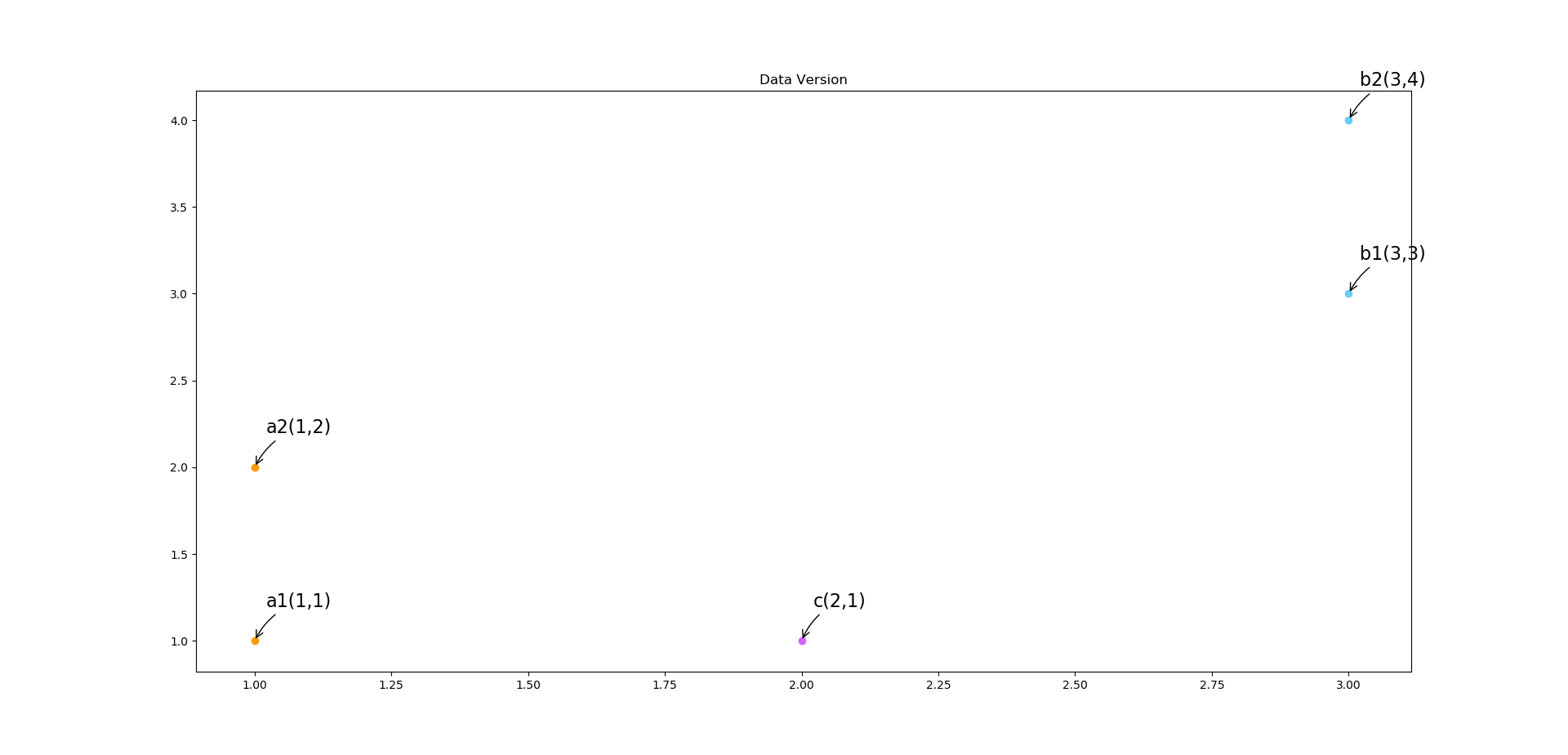1、计算测试对象与训练集中所有对象的距离，即点c与a1，a2，b1，b2的距离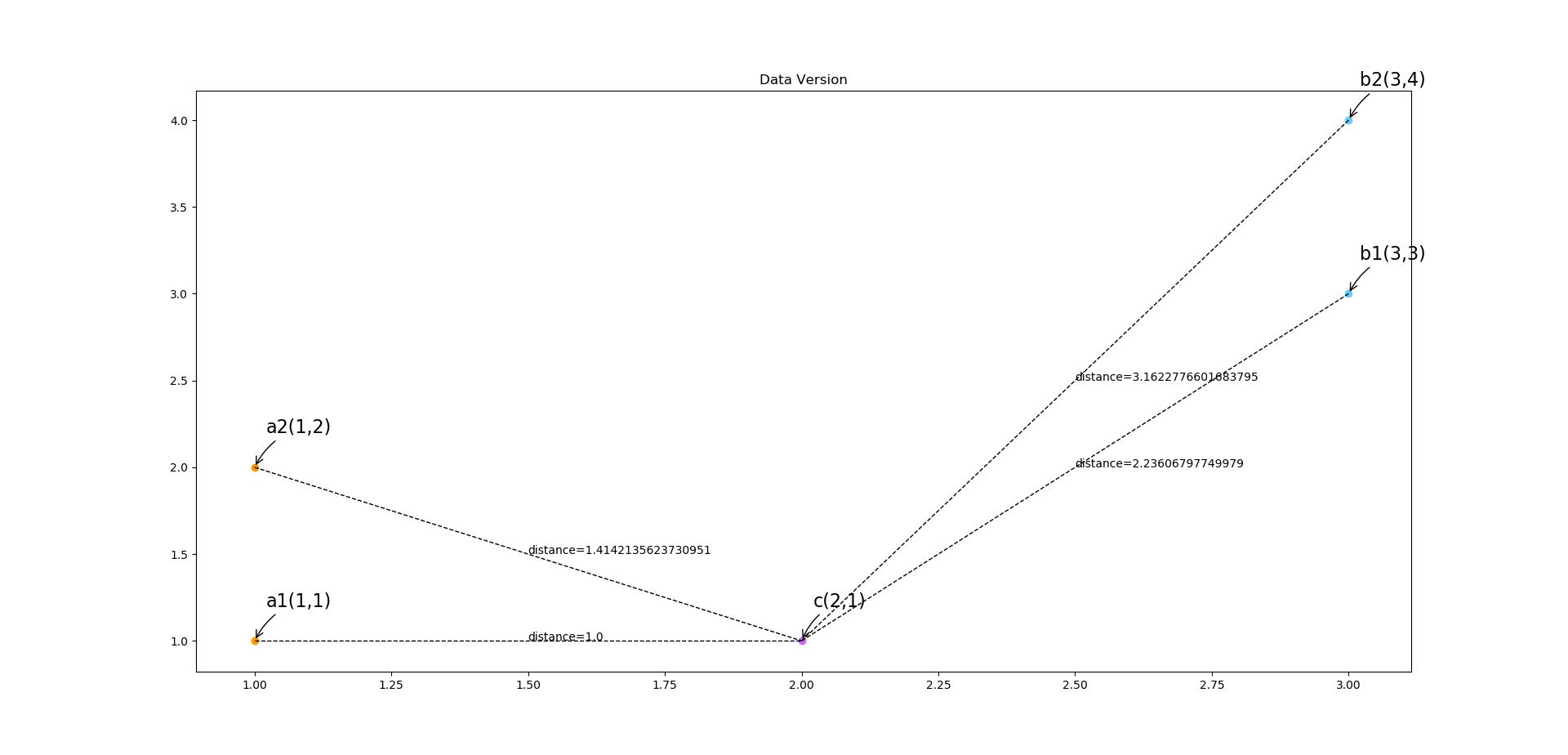2、将计算出来的距离进行升序排序

1 a1 A 1.0
2 a2 A 1.4
3 b1 B 2.2
4 b2 B 3.1

3、找出与计算对象距离最近的K个对象

1 a1 A 1.0
2 a2 A 1.4
3 b1 B 2.2

4、找出这K个对象中出现频率最高的类别，测试对象便属于该类别

# 手写数字识别系统

1、准备数据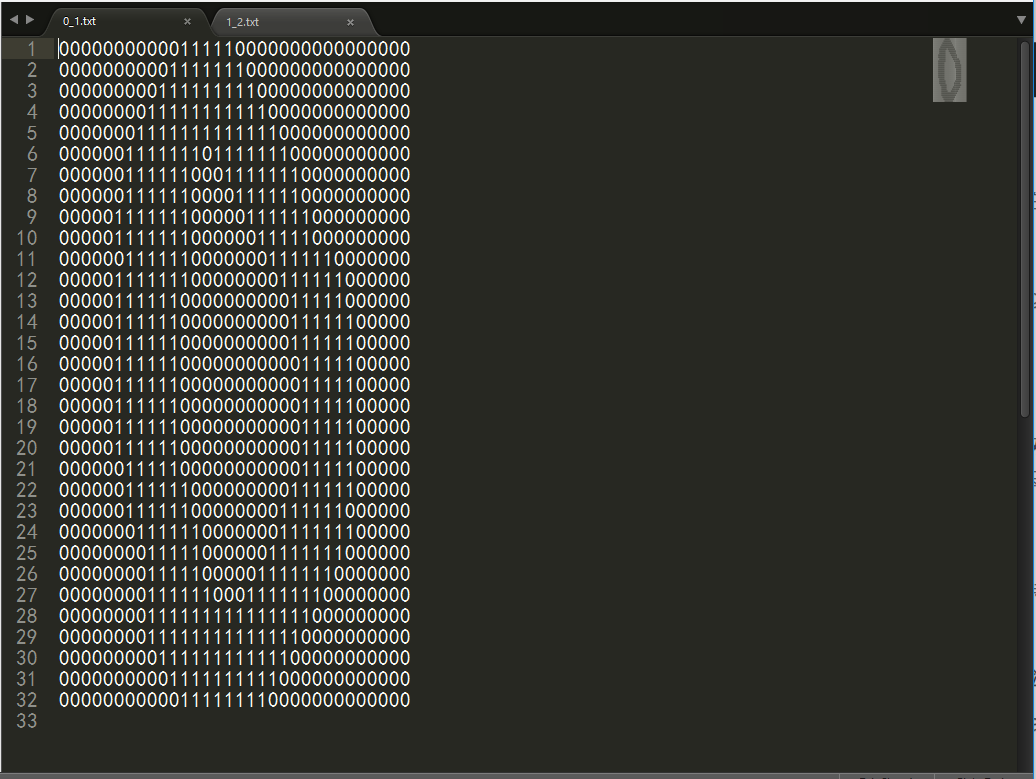2、载入数据

``````def Load_Data(file_dir):
data = []  # 数据列表
label = []  # 标签列表

file_list = os.listdir(file_dir)  # 当前目录下所有样本列表
for name in file_list:  # 读取当前目录下所有文件并转化成行向量
vector = np.zeros((1, 1024))
file_name = file_dir + "/" + name  # 样本路径
f = open(file_name)
for i in range(32):  # 转化成行向量
for j in range(32):
vector[0, i*32 + j] = int(line[j])
data.append(vector)  # 数据加入数据集
label.append([int(name.split('_'))])  # 标签加入标签集合

return np.array(data), np.array(label)
``````

``````>>> train_data, train_label = Load_Data(trian_file_dir)
>>> train_data
array([[[0., 0., 0., ..., 0., 0., 0.]],

[[0., 0., 0., ..., 0., 0., 0.]],

[[0., 0., 0., ..., 0., 0., 0.]],

...,

[[0., 0., 0., ..., 0., 0., 0.]],

[[0., 0., 0., ..., 0., 0., 0.]],

[[0., 0., 0., ..., 0., 0., 0.]]])
>>> train_label.T
array([[0, 0, 0, 0, 0, 0, 0, 0, 0, 0, 1, 1, 1, 1, 1, 1, 1, 1, 1, 1, 2, 2,
2, 2, 2, 2, 2, 2, 2, 2, 3, 3, 3, 3, 3, 3, 3, 3, 3, 3, 4, 4, 4, 4,
4, 4, 4, 4, 4, 4, 5, 5, 5, 5, 5, 5, 5, 5, 5, 5, 6, 6, 6, 6, 6, 6,
6, 6, 6, 6, 7, 7, 7, 7, 7, 7, 7, 7, 7, 7, 8, 8, 8, 8, 8, 8, 8, 8,
8, 8, 9, 9, 9, 9, 9, 9, 9, 9, 9, 9]])
``````

3、KNN算法的实现

①计算距离
②找出K个距离最近的对象
③取K个对象中类别出现频率最大一类

``````def KNN(inX, data, label, k):
"""
:param inX: 测试对象
:param data: 训练数据
:param label: 训练数据的标签
:param k: 取前k个最小值
:return:
"""

# 计算输入向量与其他样本之间的距离
squre = (data - inX)**2
SumFunction = lambda x: x.sum()
distance_squre = np.apply_along_axis(SumFunction, 2, squre)
distance = np.sqrt(distance_squre)

# 计算前K个最小值频率最高的标签
sorted_index = np.argsort(distance.T)
class_count = {}
for i in range(k):
votelabel = label[sorted_index][i]
class_count[votelabel] = class_count.get(votelabel, 0) + 1
sorted_class_count = sorted(class_count.items(), key=lambda x: x, reverse=True)

return sorted_class_count
``````

4、进行手写识别系统的测试

``````def HandWritingTest():
trian_file_dir = "../手写识别数据/trainingDigits"
test_file_dir = "../手写识别数据/testDigits"
train_data, train_label = Load_Data(trian_file_dir)  # 训练集
test_data, test_label = Load_Data(test_file_dir)  # 测试集

count = 0
for i in range(len(test_data)):
prdict = KNN(test_data[i], train_data, train_label, 3)
print("The predict label is->%s, the true label is->%s" % (prdict, test_label[i]))
if prdict == test_label[i]:
count += 1
print("The accuracy is ", count / len(test_data))
``````

``````The predict label is->0, the true label is->0
The predict label is->0, the true label is->0
The predict label is->0, the true label is->0
The predict label is->0, the true label is->0
The predict label is->0, the true label is->0
The predict label is->1, the true label is->1
The predict label is->1, the true label is->1
The predict label is->1, the true label is->1
The predict label is->1, the true label is->1
The predict label is->1, the true label is->1
The predict label is->2, the true label is->2
The predict label is->2, the true label is->2
The predict label is->2, the true label is->2
The predict label is->2, the true label is->2
The predict label is->2, the true label is->2
The predict label is->3, the true label is->3
The predict label is->3, the true label is->3
The predict label is->3, the true label is->3
The predict label is->3, the true label is->3
The predict label is->3, the true label is->3
The predict label is->4, the true label is->4
The predict label is->4, the true label is->4
The predict label is->4, the true label is->4
The predict label is->4, the true label is->4
The predict label is->4, the true label is->4
The predict label is->5, the true label is->5
The predict label is->5, the true label is->5
The predict label is->5, the true label is->5
The predict label is->5, the true label is->5
The predict label is->5, the true label is->5
The predict label is->6, the true label is->6
The predict label is->6, the true label is->6
The predict label is->6, the true label is->6
The predict label is->6, the true label is->6
The predict label is->6, the true label is->6
The predict label is->7, the true label is->7
The predict label is->7, the true label is->7
The predict label is->7, the true label is->7
The predict label is->7, the true label is->7
The predict label is->7, the true label is->7
The predict label is->8, the true label is->8
The predict label is->8, the true label is->8
The predict label is->8, the true label is->8
The predict label is->8, the true label is->8
The predict label is->8, the true label is->8
The predict label is->9, the true label is->9
The predict label is->9, the true label is->9
The predict label is->9, the true label is->9
The predict label is->9, the true label is->9
The predict label is->9, the true label is->9
The accuracy is  1.0
``````

2016-05-22 15:47:54 BaiHuaXiu123 阅读数 77113
• ###### 机器学习--线性回归数学推导视频教学

线性回归数学推导是机器学习的基石，是人工智能的算法基础， 可以被广泛的应用在在工作和学习。本节课就带领同学们从零开始一步一步的推导线性回归。课程内容包括矩阵转换、误差值分析、似然函数、小二乘、结果推导。 任务作业： 设计并实现一个三层架构的员工管理系统。要求： 1、数据库及其表自行设计，遵循数据库及表设计的一般命名规则 2、要求有管理员的登录功能及对员工信息的增删改查 3、功能的实现严格采用三层架构的设计框架，注意命名的一般规范 4、采用B/S架构，界面大方美观 5、思考下三层架构与MVC框架有什么区别 （温馨提示： 注意 作业需写在CSDN博客中，请把作业链接贴在评论区，老师会定期逐个批改~~）

3970 人正在学习 去看看 陆永剑## 摘要## 学习方式

### 监督式学习：### 非监督式学习：### 半监督式学习：### 强化学习：## 算法类似性

### 回归算法：## 基于实例的算法## 正则化方法## 决策树学习## 贝叶斯方法## 基于核的算法## 聚类算法## 关联规则学习## 人工神经网络## 深度学习## 降低维度算法### 集成算法：## 结束

2019-09-23 13:14:35 java_java38 阅读数 38
• ###### 机器学习--线性回归数学推导视频教学

线性回归数学推导是机器学习的基石，是人工智能的算法基础， 可以被广泛的应用在在工作和学习。本节课就带领同学们从零开始一步一步的推导线性回归。课程内容包括矩阵转换、误差值分析、似然函数、小二乘、结果推导。 任务作业： 设计并实现一个三层架构的员工管理系统。要求： 1、数据库及其表自行设计，遵循数据库及表设计的一般命名规则 2、要求有管理员的登录功能及对员工信息的增删改查 3、功能的实现严格采用三层架构的设计框架，注意命名的一般规范 4、采用B/S架构，界面大方美观 5、思考下三层架构与MVC框架有什么区别 （温馨提示： 注意 作业需写在CSDN博客中，请把作业链接贴在评论区，老师会定期逐个批改~~）

3970 人正在学习 去看看 陆永剑

title: 机器学习-KNN算法
date: 2019-08-16 15:17:00
categories:

• 技术
tags:
• 机器学习
• Numpy

KNN算法的理解。

#### KNN算法流程总结

``````1）计算已知类别数据集中的点与当前点之间的距离
2）按距离递增次序排序
3）选取与当前点距离最小的k个点
4）统计前k个点所在的类别出现的频率
5）返回前k个点出现频率最高的类别作为当前点的预测分类
``````
``````from sklearn.neighbors import KNeighborsClassifier

# achieve data
x = [,,,[-1],[-4]]
y = [1,1,1,0,-1]

estimator = KNeighborsClassifier(n_neighbors=3)
estimator.fit(x,y)
result = estimator.predict([[-3]])
print(result)
``````
``````[-1]
``````

#### 问题一：距离选取

1.距离公式，除了欧式距离，还有哪些距离公式可以使用？

``````（1）欧式距离（两点距离问题）：两个点在空间中的距离。
（2）曼哈顿距离（城市街区问题）：在曼哈顿街区要从一个十字路口开车到另一个十字路口，驾驶距离显然不是两点间的直线距离。这个实际驾驶距离就是“曼哈顿距离”。曼哈顿距离也称为“城市街区距离”(City Block distance)。
d(i,j)=|X1-X2|+|Y1-Y2|
（3）切比雪夫距离 (Chebyshev Distance)：国际象棋中，国王可以直行、横行、斜行，所以国王走一步可以移动到相邻8个方格中的任意一个。国王从格子(x1,y1)走到格子(x2,y2)最少需要多少步？这个距离就叫切比雪夫距离。在公式里体现就是某个维度上的最大距离。
（4）闵可夫斯基距离(Minkowski Distance)：闵氏距离不是一种距离，而是一组距离的定义，是对多个距离度量公式的概括性的表述。

``````

1 闵氏距离，包括曼哈顿距离、欧氏距离和切比雪夫距离都存在明显的缺点:
e.g. 二维样本(身高[单位:cm],体重[单位:kg]),现有三个样本：a(180,50)，b(190,50)，c(180,60)。

a与b的闵氏距离（无论是曼哈顿距离、欧氏距离或切比雪夫距离）等于a与c的闵氏距离。但实际上身高的10cm并不能和体重的10kg划等号。

`````` (1)将各个分量的量纲(scale)，也就是“单位”相同的看待了;
​ (2)未考虑各个分量的分布（期望，方差等）可能是不同的。
``````

#### 改进

``````标准化欧氏距离 (Standardized EuclideanDistance)：标准化欧氏距离是针对欧氏距离的缺点而作的一种改进。

``````

#### 其他距离

``````(1)余弦距离(Cosine Distance):

(2)汉明距离(Hamming Distance):

(3)杰卡德距离(Jaccard Distance):

（4）马氏距离(Mahalanobis Distance):马氏距离是由印度统计学家马哈拉诺比斯提出的，表示数据的协方差距离。它是一种有效的计算两个位置样本集的相似度的方法。马氏距离也可以定义为两个服从同一分布并且其协方差矩阵为∑的随机变量的差异程度：如果协方差矩阵为单位矩阵，马氏距离就简化为欧式距离；如果协方差矩阵为对角矩阵，则其也可称为正规化的欧式距离。

1.量纲无关，排除变量之间的相关性的干扰；

2.马氏距离的计算是建立在总体样本的基础上的，如果拿同样的两个样本，放入两个不同的总体中，最后计算得出的两个样本间的马氏距离通常是不相同的，除非这两个总体的协方差矩阵碰巧相同；

3 .计算马氏距离过程中，要求总体样本数大于样本的维数，否则得到的总体样本协方差矩阵逆矩阵不存在，这种情况下，用欧式距离计算即可。

4.还有一种情况，满足了条件总体样本数大于样本的维数，但是协方差矩阵的逆矩阵仍然不存在，比如三个样本点（3，4），（5，6），（7，8），这种情况是因为这三个样本在其所处的二维空间平面内共线。这种情况下，也采用欧式距离计算。

``````

#### 问题二：K值的选取

2.选取K值的大小？

``````K值过小：

k值过大：

``````
##### 解决方法

``````K值选择问题，李航博士的一书「统计学习方法」上所说：

1) 选择较小的K值，就相当于用较小的领域中的训练实例进行预测，“学习”近似误差会减小，只有与输入实例较近或相似的训练实例才会对预测结果起作用，与此同时带来的问题是“学习”的估计误差会增大，换句话说，K值的减小就意味着整体模型变得复杂，容易发生过拟合；

2) 选择较大的K值，就相当于用较大领域中的训练实例进行预测，其优点是可以减少学习的估计误差，但缺点是学习的近似误差会增大。这时候，与输入实例较远（不相似的）训练实例也会对预测器作用，使预测发生错误，且K值的增大就意味着整体的模型变得简单。

3) K=N（N为训练样本个数），则完全不足取，因为此时无论输入实例是什么，都只是简单的预测它属于在训练实例中最多的类，模型过于简单，忽略了训练实例中大量有用信息。

``````

#### 估计误差：

``````"""
1.获取数据集
2，数据基本处理
3. 特征工程
4. 机器学习（模型训练）
5. 模型评估
"""

from sklearn.model_selection import train_test_split
from sklearn.preprocessing import StandardScaler
from sklearn.neighbors import KNeighborsClassifier

# 1.获取数据集
# 2，数据基本处理
# 2.1 数据分割
x_train, x_test, y_train, y_test = train_test_split(iris.data, iris.target, random_state=23, test_size=0.1)

# 3. 特征工程
# 3.1 实例化一个转化器
transfer = StandardScaler()
# 3.2 调用fit_transform方法
x_train = transfer.fit_transform(x_train)
x_test = transfer.fit_transform(x_test)

# 4. 机器学习（模型训练）
# 4.1 实例化一个估计器
estimator = KNeighborsClassifier(n_neighbors=5)
# 4.2 模型训练
estimator.fit(x_train,y_train)
# 5. 模型评估
# 5.1 输出预测值
y_pre = estimator.predict(x_test)
print("预测值是：\n", y_pre)

# 5.2 输出准确率
ret = estimator.score(x_test, y_test)
print("准确率：\n", ret)
``````

#### KNN 算法总结

##### 优点
• 简单有效
• 重新训练代价低
• 适合类域交叉样本：KNN方法主要靠周围有限的邻近的样本,而不是靠判别类域的方法来确定所属类别的，因此对于类域的交叉或重叠较多的待分样本集来说，KNN方法较其他方法更为适合。
• 该算法比较适用于样本容量比较大的类域的自动分类，而那些样本容量较小的类域采用这种算法比较容易产生误分。
##### 缺点
• 惰性算法
• 类别评分不是规格化
• 对不均衡的样本不擅长
当样本不平衡时，如一个类的样本容量很大，而其他类样本容量很小时，有可能导致当输入一个新样本时，该样本的K个邻居中大容量类的样本占多数。该算法只计算“最近的”邻居样本，某一类的样本数量很大，那么或者这类样本并不接近目标样本，或者这类样本很靠近目标样本。无论怎样，数量并不能影响运行结果。可以采用权值的方法（和该样本距离小的邻居权值大）来改进。
• 计算量较大
目前常用的解决方法是事先对已知样本点进行剪辑，事先去除对分类作用不大的样本。

#### 【引入】交叉验证&网格搜索

##### 交叉验证

``````训练集：训练集+验证集

``````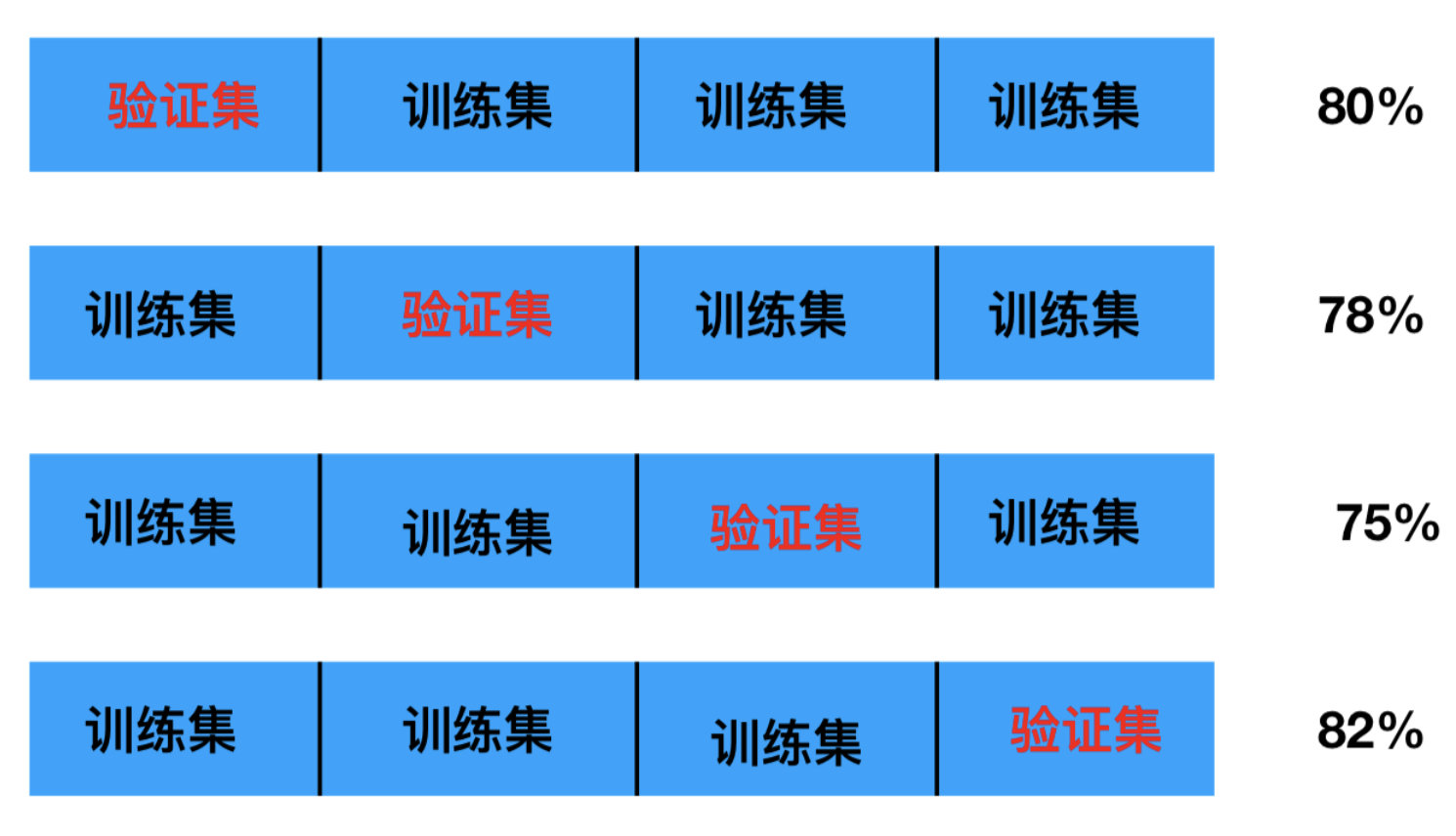##### 网格搜索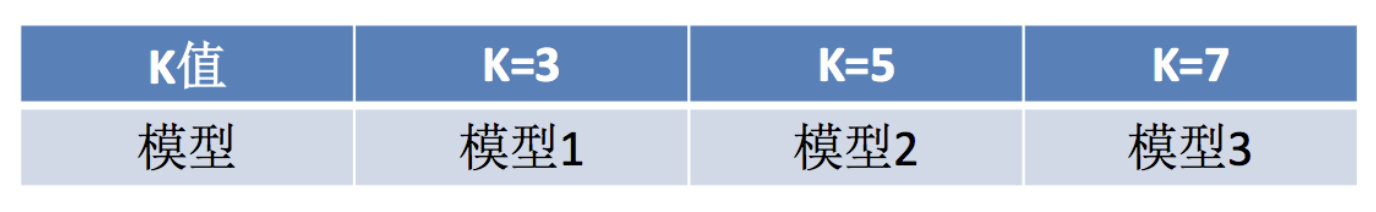##### 代码实现
``````sklearn.model_selection.GridSearchCV(estimator, param_grid=None,cv=None)
estimator -- 选择了哪个训练模型
param_grid -- 需要传递的超参数
cv -- 几折交叉验证
``````
``````"""
1.获取数据集
2，数据基本处理
3. 特征工程
4. 机器学习（模型训练）
5. 模型评估
"""

from sklearn.model_selection import train_test_split, GridSearchCV
from sklearn.preprocessing import StandardScaler
from sklearn.neighbors import KNeighborsClassifier

# 1.获取数据集
# 2，数据基本处理
# 2.1 数据分割
x_train, x_test, y_train, y_test = train_test_split(iris.data, iris.target, test_size=0.2)

# 3. 特征工程
# 3.1 实例化一个转化器
transfer = StandardScaler()
# 3.2 调用fit_transform方法
x_train = transfer.fit_transform(x_train)
x_test = transfer.fit_transform(x_test)

# 4. 机器学习（模型训练）
# 4.1 实例化一个估计器
estimator = KNeighborsClassifier(n_neighbors=1)

# 4.2 调用交叉验证网格搜索模型
param_grid = {"n_neighbors": [1, 3, 5, 7, 9]}
estimator = GridSearchCV(estimator, param_grid=param_grid, cv=10, n_jobs=4)

# 4.3 模型训练
estimator.fit(x_train, y_train)
# 5. 模型评估
# 5.1 输出预测值
y_pre = estimator.predict(x_test)
print("预测值是：\n", y_pre)

# 5.2 输出准确率
ret = estimator.score(x_test, y_test)
print("准确率：\n", ret)

# 5.3 其他平均指标
print("最好的模型:\n", estimator.best_estimator_)
print("最好的结果:\n", estimator.best_score_)
print("整体模型结果:\n", estimator.cv_results_)

``````

2017-09-05 16:06:02 iloveyousunna 阅读数 655
• ###### 机器学习--线性回归数学推导视频教学

线性回归数学推导是机器学习的基石，是人工智能的算法基础， 可以被广泛的应用在在工作和学习。本节课就带领同学们从零开始一步一步的推导线性回归。课程内容包括矩阵转换、误差值分析、似然函数、小二乘、结果推导。 任务作业： 设计并实现一个三层架构的员工管理系统。要求： 1、数据库及其表自行设计，遵循数据库及表设计的一般命名规则 2、要求有管理员的登录功能及对员工信息的增删改查 3、功能的实现严格采用三层架构的设计框架，注意命名的一般规范 4、采用B/S架构，界面大方美观 5、思考下三层架构与MVC框架有什么区别 （温馨提示： 注意 作业需写在CSDN博客中，请把作业链接贴在评论区，老师会定期逐个批改~~）

3970 人正在学习 去看看 陆永剑

# 机器学习之KNN算法python实现

## 一. 理论基础

#### 2. k值选择

• k值越大，意味着模型越简单，学习近似误差大，估计误差小，欠拟合；
• k值越小，意味着模型越复杂，学习近似误差小，估计误差大，过拟合，而且对近邻的实例点敏感.

#### 4. kd树

kd树更适用于训练实例数远大于空间维数时的k近邻搜索，当空间维数接近训练实例数时，它的效率会迅速下降，几乎接近线性扫描。

## 二. python实现

#### 1. 代码

knn.py

``````#encoding=utf-8

'''
implement the knn algorithm
'''

import numpy as np
import pandas as pd
from sklearn.model_selection import train_test_split
from scipy.stats import mode
import matplotlib.pyplot as plt

class KNN:

def __init__(self):
pass

def predict(self, x_train, y_train, x_test, k=3):
self.k = k
m_train = x_train.shape
m_test = x_test.shape
x_train = np.mat(x_train)
y_train = np.mat(y_train)
x_test = np.mat(x_test)

#1. get the distances between each sample in train samples and each sample in test samples,
#the distances matrix's shape is (m_test, m_train).
dists = self.__distance__(x_train, x_test)
#2. sort the distances by row, and get the sort index
sort_idx = np.argsort(dists, axis=1)
#3. get the x index and y index, which is top k distance sample index
x_idx = np.tile(np.mat(range(m_test)).T, [1, self.k])
y_idx = sort_idx[:, 0 : self.k]
#4. get the top k distance labels, and the matrix's shape is (m_test, k)
labels = np.tile(y_train.T, [m_test, 1])
p_labels = labels[x_idx, y_idx]
#5. get the mode of each row, which means the most labels
y_predict = np.mat(mode(p_labels, axis=1))
return y_predict

def __distance__(self, x_train, x_test):
'''
force compute to get the distance between each sample in train samples and each sample in test samples
'''
m_train = x_train.shape
m_test = x_test.shape
dists = np.zeros((m_test, m_train))
count = 0
for test in x_test:
test =  np.tile(test, [m_train, 1])
distance = np.sum(np.multiply(x_train - test, x_train - test), axis=1)
dists[count] = distance.T
count += 1
return dists

def create_kd_tree(self, datalist):
'''
create KD tree
Args:
data: data list
'''
root = KDNode()
self.build_tree(root, datalist)
self.kd_tree = root
return root

def build_tree(self, parent, datalist):
'''
recursive build tree function
Args:
parent: parent node
'''
m = datalist.shape
#if the length of data is equal to 1, the node is a leaf node
if m == 1:
parent.data = datalist
return

#compute the best split demension by the variance of each demension of the data
demension = np.argmax(np.var(datalist, axis=0))
#sort the data by the chosen demension
sorted_index = np.argsort(datalist[:, demension], axis=0)
#get the index of the middle value in the datalist
middle = m / 2
#get the left data
l_data = datalist[np.squeeze(sorted_index[0 : middle].getA()), :]
#get the right data
r_data = datalist[np.squeeze(sorted_index[middle + 1 : ].getA()), :]

#assign the property of the parent node
parent.data = datalist[np.squeeze(sorted_index[middle, :].getA())]
parent.demension = demension
parent.split_value = datalist[np.squeeze(sorted_index[middle, :].getA()), demension]

#recursive build the child node if the length of rest data is not equal to zero
if len(l_data) != 0:
l_node = KDNode()
parent.left = l_node
self.build_tree(l_node, l_data)

if len(r_data) != 0:
r_node = KDNode()
parent.right = r_node
self.build_tree(r_node, r_data)

def __distance_by_kd_tree__(self, x_test):
'''
get nearest neighbors matrix by kd_tree search
'''
m = x_test.shape
dists = np.zeros((m, 1))
count = 0
for x in x_test:
dists[count] = self.__find_neighbor__(x, self.kd_tree)
count += 1
return np.mat(dists)

def __find_neighbor__(self, x, node):
'''
recursive find the neighbor of x in kd-tree
Args:
the root node of current child tree

steps:
1. if the current is leaf node, return the data in the node as the nearest neighbor
2. if the value of x is less than the split value, take the neighbor of left child
tree as nearest neighbor. And then check if another child tree has the more nearest
neighbor;
if the value of x is more than the split value, do it as like mentioned above;
3. check if the current node and x has more nearest distance
'''

if node.demension == None:
return node.data

if (x[0, node.demension] <= node.split_value) and node.left:
neighbor = self.__find_neighbor__(x, node.left)
if node.right \
and (np.abs(x[0, node.demension] - node.split_value) < self.__euclidean_distance__(x, neighbor)) \
and (self.__euclidean_distance__(self.__find_neighbor__(x, node.right), x) < self.__euclidean_distance__(x, neighbor)):
neighbor = self.__find_neighbor__(x, node.right)
elif (x[0, node.demension] > node.split_value) and node.right:
neighbor = self.__find_neighbor__(x, node.right)
if node.left \
and (np.abs(x[0, node.demension] - node.split_value) < self.__euclidean_distance__(x, neighbor)) \
and (self.__euclidean_distance__(self.__find_neighbor__(x, node.left), x) < self.__euclidean_distance__(x, neighbor)):
neighbor = self.__find_neighbor__(x, node.left)
else:
# this happens as like:
# x = 6, node = 5
#         5
#        /
#       4
neighbor = node.data

if self.__euclidean_distance__(x, node.data) < self.__euclidean_distance__(x, neighbor):
neighbor = node.data
return neighbor

def __euclidean_distance__(self, x1, x2):
'''
compute the euclidean distance
'''
return np.sum(np.multiply(x1 - x2, x1 - x2))

class KDNode:
def __init__(self, data=None, demension=None, split_value=None, left=None, right=None):
self.data = data
self.demension = demension
self.split_value = split_value
self.left = left
self.right = right

def main():
'''
KNN test unit
'''

data['label'] = data['label'] + 1
x_train, x_test, y_train, y_test = train_test_split(
data.values[:, 0:2],
data.values[:, 2],
test_size=0.2,
random_state=0
)

x_train = np.mat(x_train)
x_test = np.mat(x_test)
y_train = np.mat(y_train).T
y_test = np.mat(y_test).T

#2. predict
print '2. predicting...'
knn = KNN()
y_predict = knn.predict(x_train, y_train, x_test, k=1)

#3. show the results
print '3. show the results...'
plt.scatter(x_train.getA()[:, 0], x_train.getA()[:, 1], c=y_train.T.getA(), marker='o')
plt.scatter(x_test.getA()[:, 0], x_test.getA()[:, 1], c=y_predict.T.getA(), marker='*')
plt.show()

def test_build_tree():
'''
test building the kd tree
'''
datalist = np.mat([[3, 1, 4],
[2, 3, 7],
[2, 1, 3],
[2, 4, 5],
[1, 4, 4],
[0, 5, 7],
[6, 1, 4],
[4, 3, 4],
[5, 2, 5],
[4, 0, 6],
[7, 1, 6]])
knn = KNN()
tree = knn.create_kd_tree(datalist)
res = knn.__find_neighbor__(np.mat([[3,1,5]]), tree)
print res

if __name__ == '__main__':
main()
``````

#### 2. 结果#### 3. 数据

``````x,y,label
14.7,17.85,0
17.45,17.45,0
18.85,15.15,0
17.25,13.7,0
13.9,12.5,0
10.5,15.65,0
8.4,20.5,0
11.1,21.85,0
17.6,21.65,0
23.0,19.75,0
24.45,12.4,0
16.25,3.3,0
8.85,5.05,0
5.55,8.8,0
6.05,11.75,0
26.45,6.9,0
28.95,6.6,0
21.75,8.35,0
21.05,10.95,0
23.9,17.05,0
19.7,18.2,0
12.4,19.3,0
9.25,18.4,0
10.3,8.95,0
16.65,8.6,0
37.3,15.1,0
32.5,10.0,1
33.05,11.45,1
25.75,17.1,1
20.15,17.8,1
12.85,20.75,1
12.8,8.65,1
14.65,5.6,1
24.2,6.3,1
24.1,11.45,1
22.05,10.85,1
17.2,12.85,1
13.7,15.55,1
6.4,19.45,1
8.1,11.5,1
14.9,10.35,1
10.05,12.65,1
25.3,1.55,1
16.5,3.8,1
17.0,6.25,1
17.85,7.35,1
23.75,9.7,1
21.65,16.3,1
16.3,19.8,1
13.9,19.85,1
13.1,14.35,1
16.55,17.9,1
16.3,18.15,1
15.3,17.7,1
13.35,18.3,1
12.8,17.5,1
13.9,15.65,1
15.65,16.5,1
31.35,7.2,1
31.35,6.95,1
29.45,5.6,1
27.15,4.85,1
26.6,5.2,1
27.8,7.35,1
28.7,8.35,1
28.8,10.25,1
5.65,11.25,1
7.8,9.7,1
7.5,11.9,1
3.55,14.45,1
3.5,13.65,1
5.1,10.95,1
5.1,11.05,1
18.65,9.1,2
19.4,10.95,2
20.1,12.7,2
17.25,14.85,2
14.6,15.25,2
14.8,11.75,2
13.5,6.4,2
14.75,5.25,2
18.05,4.05,2
21.25,3.3,2
23.75,3.85,2
32.65,5.5,2
33.65,7.05,2
32.15,13.2,2
30.8,15.25,2
30.15,16.5,2
24.7,18.0,2
22.05,19.45,2
20.1,21.5,2
20.0,22.05,2
26.8,22.45,2
29.7,21.8,2
30.95,21.35,2
30.85,19.15,2
28.4,18.7,2
26.35,19.65,2
26.5,19.9,2
30.05,19.35,2
32.75,16.35,2
33.95,14.65,2
34.05,14.6,2
30.05,18.3,3
27.65,20.6,3
25.05,21.85,3
24.1,18.2,3
23.8,15.3,3
25.6,14.45,3
28.1,12.4,3
29.35,10.95,3
29.85,8.25,3
30.55,14.1,3
28.45,15.7,3
31.85,18.15,3
18.2,19.3,3
16.85,19.8,3
7.45,9.35,3
13.35,13.9,3
32.4,9.75,3
23.8,1.05,3
30.75,4.05,4
30.5,5.3,4
30.35,5.95,4
28.9,9.0,4
27.7,9.9,4
24.75,11.4,4
21.65,13.8,4
19.75,17.45,4
23.4,20.05,4
18.2,21.75,4
9.65,18.4,4
5.6,13.45,4
8.8,9.75,4
11.25,11.2,4
5.35,15.95,4
6.1,16.0,4
24.25,15.95,4
31.55,17.0,4
32.45,14.0,4
24.05,12.4,4
12.3,12.85,4
7.15,19.3,4
21.35,22.4,4
27.95,17.65,4
24.3,7.7,4
17.5,3.6,4
12.7,6.95,4
11.25,10.7,4
9.0,15.2,4
7.05,19.15,4
17.45,13.4,4
16.0,10.75,4
16.75,12.0,4
18.25,11.5,4
18.15,9.15,4
17.1,9.5,4
17.0,10.25,4
12.8,7.75,4
17.0,6.7,4
21.15,8.5,4
20.35,9.35,4
19.45,10.0,4
18.45,10.05,4
18.0,8.0,4
20.15,8.0,4
21.45,6.65,4
19.2,6.45,4
15.25,8.4,4
14.8,9.5,4
14.45,7.7,4
16.45,6.6,4
18.0,5.85,4
18.85,5.7,4
19.6,6.1,4
29.9,14.15,6
31.4,15.8,6
32.15,15.3,6
33.25,13.65,6
33.8,11.95,6
33.85,10.9,6
33.9,10.35,6
32.6,10.75,6
32.1,12.55,6
34.15,12.55,6
35.35,11.8,6
35.15,10.4,6
34.65,9.1,6
34.3,8.9,6
35.55,9.25,6
36.35,12.45,6
37.75,9.4,6
37.75,8.5,6
36.4,8.2,6
35.0,8.05,6
35.65,7.15,6
37.55,6.4,6
39.2,7.1,6
36.5,9.85,0
36.8,9.35,0
37.5,7.7,0
34.05,9.8,0
20.2,20.3,0
26.45,21.1,0
27.9,20.65,0
27.15,16.9,0
25.5,13.1,0
24.05,10.2,0
23.45,5.3,0
20.8,10.95,0
18.95,14.65,0
17.15,16.25,0
11.3,17.0,0
11.65,11.1,0
15.95,4.8,0
21.45,3.25,0
13.9,3.05,0
10.75,6.2,0
9.3,16.85,0
10.25,19.5,0
12.7,15.95,0
13.3,14.3,0
15.7,11.45,0
16.1,10.9,0
14.1,14.2,0
14.35,13.65,0
15.3,14.1,0
15.65,14.7,0
15.75,15.85,0
15.75,15.85,0
19.2,18.4,0
19.2,17.05,0
19.3,15.6,0
20.45,14.1,0
21.4,11.65,0
26.3,11.85,2
20.5,18.75,2
17.55,19.95,2
13.1,16.65,2
9.55,12.7,2
7.85,15.65,2
7.75,16.8,2
9.1,10.35,2
21.25,8.6,2
22.65,5.0,2
11.75,10.7,1
11.05,14.55,1
13.85,8.45,1
11.7,6.65,1
10.75,4.95,1
10.95,3.75,1
6.85,8.35,1
11.35,5.7,1
13.25,4.6,1
7.45,7.95,1
15.7,13.35,1
16.85,14.3,1
13.55,10.4,1
9.55,7.3,1
34.3,8.0,1
28.15,8.45,1
25.15,8.75,1
22.3,14.6,1
29.5,15.55,1
28.2,14.1,1
32.95,10.15,1
29.15,11.4,1
20.85,18.95,1
22.0,17.8,1
12.5,2.35,2
7.25,14.5,3
6.1,18.25,3
8.85,20.5,3
7.55,22.25,3
15.8,19.2,3
16.2,18.0,3
16.95,17.45,3
17.35,18.2,3
18.25,17.45,3
17.85,17.15,3
18.25,16.25,3
19.75,16.15,3
20.85,16.95,3
21.8,17.95,3
22.75,19.0,3
24.2,19.15,3
25.05,18.55,3
25.25,17.45,3
13.95,11.1,3
15.7,10.0,3
14.3,9.85,3
14.3,10.65,3
14.85,11.1,3
10.9,7.9,3
9.1,8.5,3
11.55,16.5,2
11.05,18.5,2
20.4,7.5,1
19.95,8.7,1
27.55,2.05,4
26.6,2.5,4
27.05,3.65,4
28.65,4.1,4
30.6,11.8,4
29.55,12.35,4
29.0,13.05,4
30.5,13.4,4
31.6,14.05,4
31.55,14.9,4
28.6,17.1,4
30.35,17.75,4
34.7,12.25,4
31.0,11.75,4
16.0,12.7,4
8.8,17.95,4
14.45,3.7,4
28.6,4.75,4
29.7,10.85,4
24.15,14.2,4
14.85,12.0,4
6.9,11.05,4``````

2016-05-01 15:45:22 chenchunyue11 阅读数 4919
• ###### 机器学习--线性回归数学推导视频教学

线性回归数学推导是机器学习的基石，是人工智能的算法基础， 可以被广泛的应用在在工作和学习。本节课就带领同学们从零开始一步一步的推导线性回归。课程内容包括矩阵转换、误差值分析、似然函数、小二乘、结果推导。 任务作业： 设计并实现一个三层架构的员工管理系统。要求： 1、数据库及其表自行设计，遵循数据库及表设计的一般命名规则 2、要求有管理员的登录功能及对员工信息的增删改查 3、功能的实现严格采用三层架构的设计框架，注意命名的一般规范 4、采用B/S架构，界面大方美观 5、思考下三层架构与MVC框架有什么区别 （温馨提示： 注意 作业需写在CSDN博客中，请把作业链接贴在评论区，老师会定期逐个批改~~）

3970 人正在学习 去看看 陆永剑

# 机器学习常见的优化算法

## 1、梯度下降法alpha为梯度下降的学习率，也称步长。## 2、牛顿法（拟牛顿法）

（1）求解方程的牛顿法（2）牛顿方法求最优化问题（3）拟牛顿算法

x(k+1)-x(k)=(f'(k+1)-f'(k))/H，因此，G满足x(k+1)-x(k)=G*(f'(k+1)-f'(k))。因此该条件为称为拟牛顿法。按照牛顿条件，在每次迭代中可以选择更新矩阵G(k+1)=G(k)+(delta)G(k)。3、拉格朗日对偶性

1.  http://blog.csdn.net/abcjennifer/article/details/7700772

2. Andrew Ng 在Machine Learning的课件

3. 李航.统计学习基础